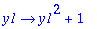Error: variables must be unique and of type name

This error occurs in unapply , which is used to make an expression into a function of one or more variables. The first argument must be the expression, and the other arguments are the variables. This error occurs if the "variable" arguments are not all names (indexed names are OK), or if two of those variables are equal.

Like most procedures, unapply begins by evaluating its arguments. Therefore if a variable has been assigned a value, it will be replaced by that value. If the value is not a name, the result will be this error.

Examples:

Second argument an expression, not a name:

> unapply((x+1)^2+1,x+1);

Error, (in unapply) variables must be unique and of type name

In this case, you could use the replacement procedure vnapply instead of unapply .

vnapply((x+1)^2+1,x+1);Variable is assigned a value:

> p:= x+1:
unapply(p^2+1,p);

Error, (in unapply) variables must be unique and of type name

This could be fixed using quotes to delay evaluation.

> unapply('p^2+1','p');Two variable arguments equal:

> unapply(x^2+1,x,x);

Error, (in unapply) variables must be unique and of type name

Maple Advisor Database R. Israel, 1998